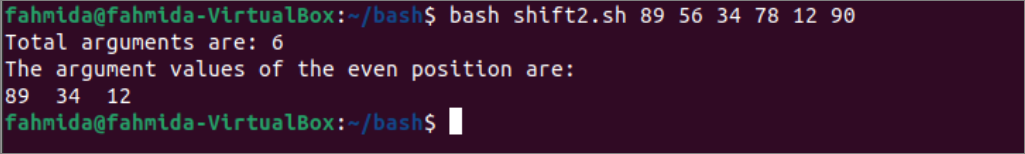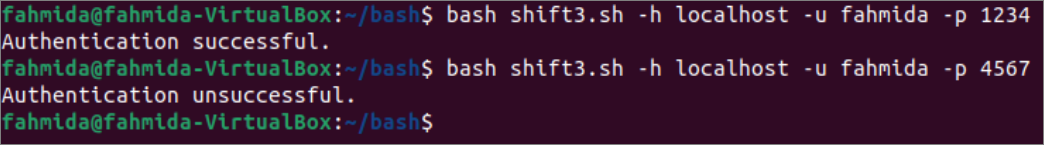Gice

Technology and General BlogWhen the command-line arguments take inputs from the user, the first argument contains the script name. Sometimes the script name is required to omit for programming purposes. This task can be done easily by using any loop in bash. Using the `shift` command is another way to do the task. This command is used to move one positional parameter to the left by default. The different uses of the `shift` command in bash have shown in this tutorial.

Syntax:

The syntax of the `shift` command is given below. This command has one optional argument that is used to set the number of positions that will be shifted to the left. The argument must be positive. If the argument value is set to 0, then no command-line argument will be shifted. If no argument is used, then one command-line argument will be shifted by default.

Example-1: Print all command-line argument values

Create a bash file with the following script to print the command line argument values using ‘for’ loop without `shift` command and ‘while’ loop with `shift` command. Each argument value will be stored in the variable, the value when the for loop will execute, and this variable will be printed later. The start variable has used in the while loop to read each argument value by using the `shift` command and terminate the loop when all command-line arguments are printed. A counter variable, i, has been used in the script to display the number of the argument. If no command-line argument is given at the execution time, then an empty string will be set for the first argument, and nothing will be printed.

#!/bin/bash
#Print the command-line aegument values using for and while loop
#Set the counter
i=1
echo “The argument values without shift command:”
#Iterate all values using for loop
for value in“[email protected]”
do
echo “Argument no. \$i = \$value
((i++))
done

#Re-initialize the counter
i=1
#Set the optional argument
start=\$1:-“”

echo “The argument values by using shift command:”
#Iterate all values using while loop
while [ \$start != “” ];
do
echo “Argument no. \$i = \$start
#Shift each argument by 1
shift
start=\$1
((i++))
done

Output:

According to the following output, the above script has been executed with three command-line argument values. The argument values are printed two times using the for loop and while loop with the `shift` command.

Example-2: Print the argument values of even position

In the previous example, no argument has used with the `shift` command, and the argument value was shifted by 1 to the left. The use of the optional argument of the `shift` command is shown in this example. Create a bash file with the following script to print the command line argument values of the even position. The total number of command-line arguments have counted and stored in the variable total, and it has been used in the while loop to iterate the loop. The `shift` command has been used with the argument value 2 to shift two command-line arguments in each iteration of the loop. Each command-line argument of the even positions will be printed with space after executing the script.

#!/bin/bash

#Count the total number of command-line arguments
total=\$#
echo “Total arguments are: \$total

echo “The argument values of the even position are:”
while [ \$total -ge0 ];
do
#Print the argument value with space
echo -n \$1 ” “
#Shift two arguments
shift 2
#Decrement the counter by 2
((total=\$total2))
done

echo

Output:

According to the following output, the above script executed six command-line argument values without the script name. After printing the value 89, the next value of the even position is 34, and the next value of the even position is 12.Example-3: Read the particular values of specific arguments

The way to read specific command line argument values using the `shift` command without using any loop has shown in this example. Create a bash file with the following script. The total number of command-line arguments has been counted in the script, and if the total value is less than 6, the script will be terminated by displaying an error message. If the six command-line arguments are given properly, the second argument will contain the hostname, the fourth argument will contain the username, and the sixth argument will contain the password. The hostname will be stored in a variable by shifting one argument left. The username will be stored in a variable by shifting two arguments left. The password will be stored in a variable by shifting two arguments left again. Next, the hostname, username, and password values will be compared with three string values to authenticate the user.

#!/bin/bash

#Count the total number of command-line arguments
total=\$#

#Check the 6 argument values are given or not
if [ \$total -lt6 ]; then
echo “Invalid number of arguments.”
else
#Shift one argument
shift 1
hostname=\$1
#Shift two arguments
shift 2
#Shift two arguments
shift 2
fi

#Check the values for the authentication
if [[ \$hostname == “localhost”&& \$username == “fahmida”&& \$password == “1234” ]]; then
echo “Authentication successful.”
else
echo “Authentication unsuccessful.”
fi

Output:

According to the output, the above script was executed two times with six argument values. In the first execution, the valid data were passed, and a success message has printed. On the second execution, invalid data were passed, and a failure message has printed.Conclusion:

The `shift` command is a very helpful command for reading particular command-line argument values. Different uses of this command have been described in this tutorial by using multiple examples to help the readers understand the purpose of using this command in bash script.